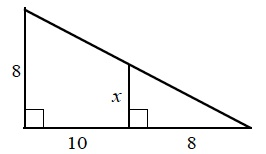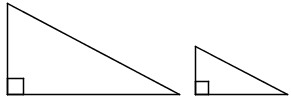### Home > AC > Chapter 6 > Lesson 6.2.5 > Problem6-84

6-84.

Examine the diagram below. The smaller triangle is similar to the larger triangle. Write and solve a proportion to find $x$.Draw two separate triangles. The larger triangle has base $10 + 8 = 18$.

$\frac{x}{8}=\frac{8}{18}$